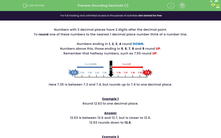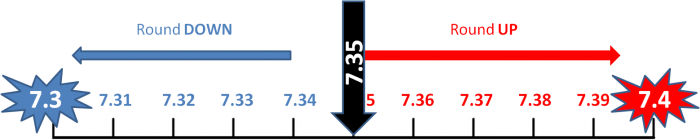# Rounding Decimals (1)

In this worksheet, students must round numbers with two decimal places to one decimal place.Key stage:  KS 2

Curriculum topic:   Number: Fractions, Decimals and Percentages

Curriculum subtopic:   Round Up/Down Decimals

Popular topics:   Estimation worksheets

Difficulty level:#### Worksheet Overview

Numbers with 2 decimal places have 2 digits after the decimal point.

To round one of these numbers to the nearest 1 decimal place number think of a number line.

Numbers ending in 1, 2, 3, 4 round DOWN.

Numbers above this, those ending in 5, 6, 7, 8 and 9 round UP.

Remember that halfway numbers, such as 7.50 round UP.Here 7.35 is between 7.3 and 7.4, but rounds up to 7.4 to one decimal place.

Example 1

Round 12.63 to one decimal place.

12.63 is between 12.6 and 12.7, but is closer to 12.6.

12.63 rounds down to 12.6.

Example 2

Round 10.38 to one decimal place.

10.38 is between 10.3 and 10.4, but is closer to 10.4.

10.38 rounds up to 10.4.

Example 3

Round 16.65 to one decimal place.

16.65 is halfway between 16.6 and 16.7 and so we round up.

16.65 rounds up to 16.7.

### What is EdPlace?

We're your National Curriculum aligned online education content provider helping each child succeed in English, maths and science from year 1 to GCSE. With an EdPlace account you’ll be able to track and measure progress, helping each child achieve their best. We build confidence and attainment by personalising each child’s learning at a level that suits them.

Get started